Function Repository Resource:

# ShuffleOrder

Determine how many shuffles restore the list of specified size to its original order

Contributed by: Wolfram Staff
 ResourceFunction["ShuffleOrder"][shuffle,n] gives the shuffle order for a size-n list.

## Details and Options

The shuffle order is the number of times a shuffle should be repeated to restore the original sequence of elements in the list.
The shuffle order is also known as the shuffle period.
In ResourceFunction["ShuffleOrder"][shuffle,n], shuffle can be one of the following:
 "type" string type, as defined in the resource function Shuffle ResourceFunction["Shuffle"][…] operator form of ResourceFunction["Shuffle"] fun a pure function that acts on a list

## Examples

### Basic Examples (2)

The shuffle order of a perfect out shuffle of a list of the specified size:

 In:=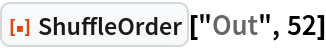Out=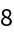Same as:

 In:=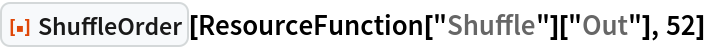Out=### Properties and Relations (2)

ShuffleOrder is determined by the PermutationOrder of a shuffle:

 In:=Out=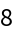In:=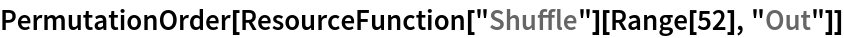Out=Or, alternatively, by the least common multiple of the lengths of its cycles:

 In:=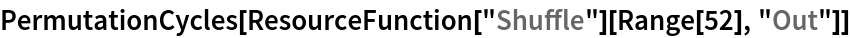Out=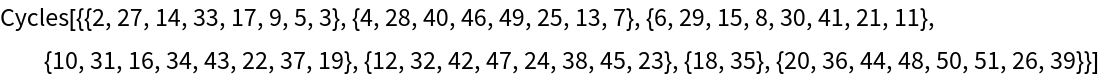In:=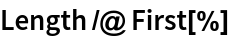Out=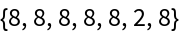In:=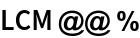Out=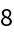The shuffle order can also be determined, possibly less efficiently, using its definition:

 In:=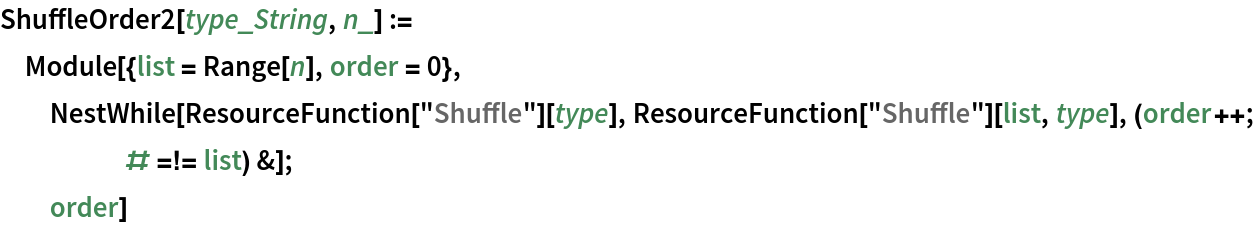For a milk shuffle:

 In:=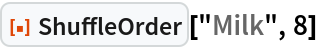Out=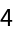In:=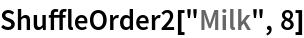Out=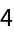### Neat Examples (1)

Restore a shuffled deck of cards to its original order:

 In:=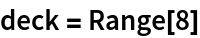Out=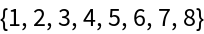In:=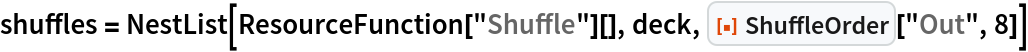Out=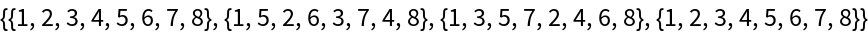In:=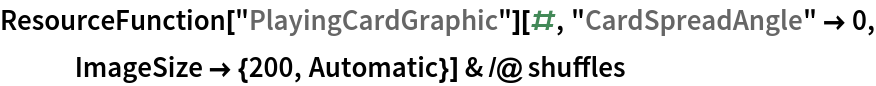Out=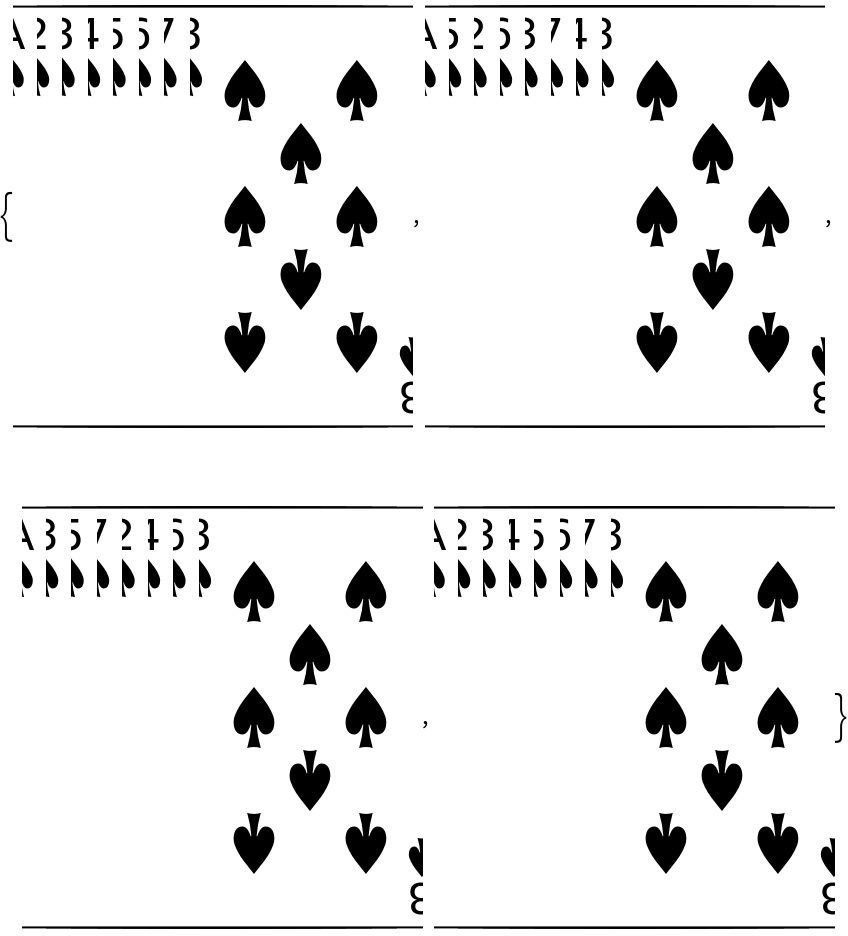## Version History

• 1.0.0 – 14 September 2020